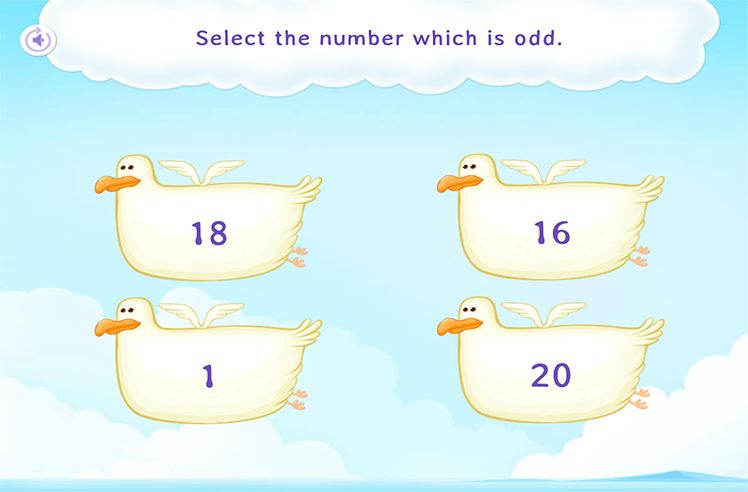# Ordinal Numbers – Definition with Examples

» Ordinal Numbers – Definition with Examples

## Ordinal Numbers

Cardinal numbers

The numbers which give us the exact quantity of an object are called cardinal numbers. In other words, cardinal numbers answer “How many?”

For instance: The given picture shows 4 cars in a parking lot.  Hence, 4 or ‘four’ here is a cardinal number.

Ordinal numbers

The numbers which give us the exact position of an object are called ordinal numbers. Ordinal numbers tell the position of an object rather than their quantity.

For instance:

Example 1- The given picture shows different floors in a building. We can use ordinal numbers to define their position.

The numbers 1st(First), 2nd(Second), 3rd(Third), 4th(Fourth), 5th(Fifth), 6th(Sixth), 7th(Seventh), 8th(Eighth), 9th(Ninth) and 10th(Tenth) tell the position of different floors in the building. Hence, all of them are ordinal numbers.

Example 2-The given picture shows some athletes competing in a 400 m race. We can use ordinal numbers to define their position.

The numbers 1st(First), 2nd(Second), 3rd(Third), 4th(Fourth), 5th(Fifth), 6th(Sixth), 7th(Seventh), 8th(Eighth), 9th(Ninth) and 10th(Tenth) tell the position of different athletes in the race. Hence, all of them are ordinal numbers.

Comparison Chart: Ordinal Numbers v/s Cardinal NumbersNumber Sequence

Play NowNumber Recognition

Play NowEven and Odd Numbers

Play Now